# From a photograph of a homeowner using a snowblower, it is determined that the radius of curvature of the trajectory of the snow was 30 ft as the snow left the discharge chute at A. Determine (a) the discharge velocity vA of the snow, (b) the radius of curvature of the trajectory at its maximum height. Fig. P11.143

Question-AnswerCategory: Engineering MechanicsFrom a photograph of a homeowner using a snowblower, it is determined that the radius of curvature of the trajectory of the snow was 30 ft as the snow left the discharge chute at A. Determine (a) the discharge velocity vA of the snow, (b) the radius of curvature of the trajectory at its maximum height. Fig. P11.143

From a photograph of a homeowner using a snowblower, it is determined that the radius of curvature of the trajectory of the snow was 30 ft as the snow left the discharge chute at A. Determine (a) the discharge velocity vA of the snow, (b) the radius of curvature of the trajectory at its maximum height.
Fig. P11.143Step: 1

a)
Show the acceleration of snow, tangential, and normal component of acceleration using vector diagram as in Figure (1).Step: 2

In Figure (1), snow is acted upon by acceleration due to gravity. Direction of tangential component of acceleration is against the direction of motion since speed of snow decreases. Direction of normal component of acceleration is acting towards the centre of curvature.
Write the normal component of acceleration vector a as: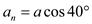…… (1)
Here, magnitude of normal component of acceleration vector isand magnitude of acceleration is a.
Choose magnitude of acceleration vector as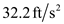.
Substitutefor a in Equation (1) to find.Thus, magnitude of normal component of acceleration vector is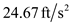.

Step: 3

Write the normal component of acceleration of an object in circular motion as the ratio between the square of the speed and radius of curvature of the path.…… (2)
Here, normal component of acceleration of object is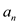, speed of the snow is v, and radius of curvature is.
Rewrite Equation (2) as: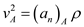…… (3)
Substitutefor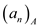and 30ft for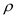in Equation (3) to find v.Hence, magnitude of discharge velocity isat an angle of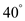.

Step: 4

b)
Write x-component of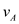as:…… (4)
Here, x-component ofis.
Substituteforin Equation (4).Thus, x-component ofis. Maximum height of the trajectory depends on the x-component ofand the only acceleration that acts on the snow is acceleration due to gravity. Thus,.

Step: 5

Rewrite Equation (2) to find the radius of curvature of trajectory at its maximum height.…… (5)
Substituteforandfor a in Equation (5).Hence, the radius of curvature of trajectory at its maximum height is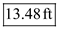.